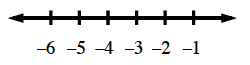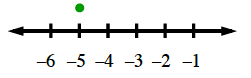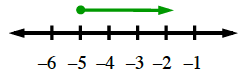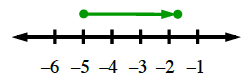Home > CC2MN > Chapter cc22 > Lesson cc22.2.2 > Problem2-53

2-53.

Use a number line to show your work as you find the value of each expression. Homework Help ✎

1. $-5+3.25$

Draw a number line.

Draw a point above the first number in the expression.

Is the number being added positive? If so, draw an arrow going in the positive direction. If the second number is negative, draw the arrow going in the negative direction. The arrow's length is the second number.

The point where the line ends is the answer.$-1.75$

2. $3.18+(−7)$

Follow the steps given in the help for part (a).